Maths-
General
Easy

Question

# The length of the tangent of the curves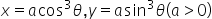is

##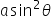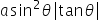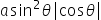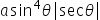Hint:

## The correct answer is:### The given values of x and y are as follows:x = acos3θy = asin3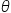The formula for length of tangent isLength =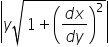We will first differentiate x and y w.r.t θ and then find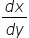Differentiating x w.r.t θDifferentiating y w.r.t θ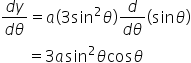Now,We will substitute this value in the formula of length of tangent.So, length of the tangent is asin2θ

For such questions, we should know the properties of trignometric functions and their different formulas.#### With Turito Foundation.#### Get an Expert Advice From Turito.